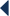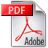Back to 3/2016
Technical Issues
3/2016 pp. 13-18

## Zastosowanie algebr hiperzespolonych w przetwarzaniu sygnałówGet full text pdf

Abstract

The subject of this paper is the application of hypercomplex algebras (in particular quaternions and octonions) in the analysis of time-invariant linear systems. We present the Cayley-Dickson construction of hypercomplex algebras and its important properties. Moreover, we formulate the concept of quaternion and octonion Fourier transform and their properties important from the signal processing point of view. We present an overview of known quaternion Fourier transform applications in the analysis of systems and partial differential equations of two variables. We also point out the direction of further work in the subject of application of the octonion Fourier transform in system analysis and analysis of partial differential equations of three variables. Such considerations are possible thanks to recently proved properties of octonion Fourier transform, which are also stated in this paper.

Key words

hypercomplex algebras, quaternion Fourier transform, octonion Fourier transform, system analysis, partial differential equations

References

1. Błaszczyk, Ł., Snopek, K.M., Symmetry properties of the Octonion Fourier Transform, Bull. Pol. Ac.: Tech. 2016, (w druku).

2. Błaszczyk, Ł., Analiza hiperzespolona w środowisku Mathematica, Zagadnienia Aktualnie Poruszane Przez Młodych Naukowców, cz. 6, 2016, (w druku)].

3. Dickson, L.E., On Quaternions and Their Generalization and the History of the Eight Square Theorem, Annals of Mathematics, 1919, 20 (3), pp. 155-171.

4. Ell, T.A., Quaternion-Fourier Transforms for Analysis of Two-Dimensional Linear Time-Invariant Partial Differential Systems, Proc 32nd IEEE Conf Decis Control, 1993, pp. 1830-1841.

5. Falcão, M.I., Miranda, F., Quaternions: A Mathematica Package for Quaternionic Analysis, Lecture Notes in Computer Science, 2011, 6784, pp. 200-214.

6. Hamilton, W.H., On quaternions, Proc. Royal Irish Academy, 1847, 3, pp. 1-16.

7. Kurman, K., Liczby podwójne zespolone i możliwość ich zastosowania. Wyk. P.W. ZPS, 1958, 245 (16).

8. Snopek, K., Studies on Complex and Hypercomplex Multidimensional Analytic Signals, Zeszyty Naukowe serii Elektronika, z. 190, Oficyna Wydawnicza PW, Warszawa, 2013.

9. Weinberger, H.F., A First Course in Partial Differential Equations with Complex Variables and Transform Methods, John Wiley & Sons, 1965.